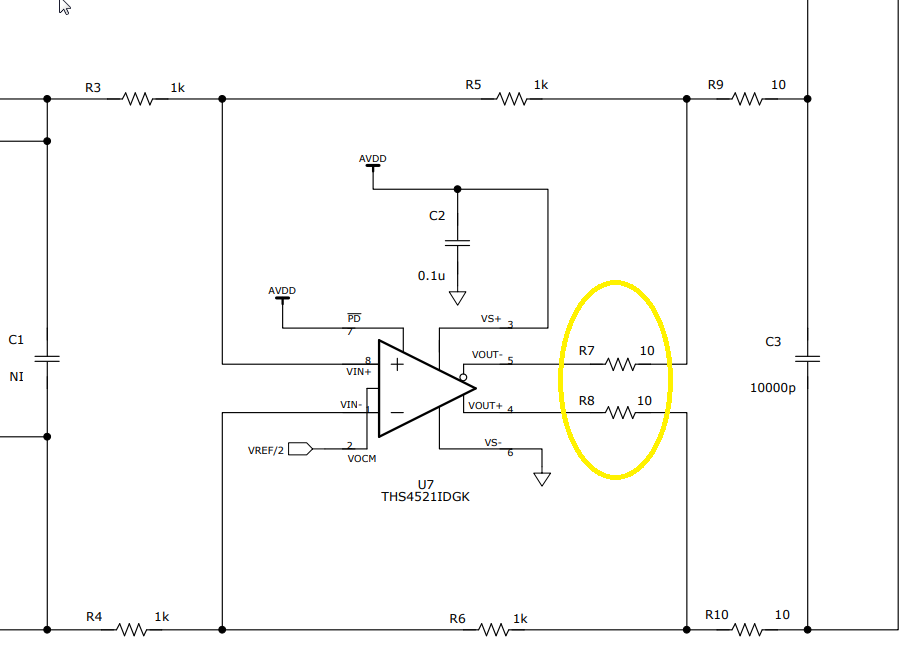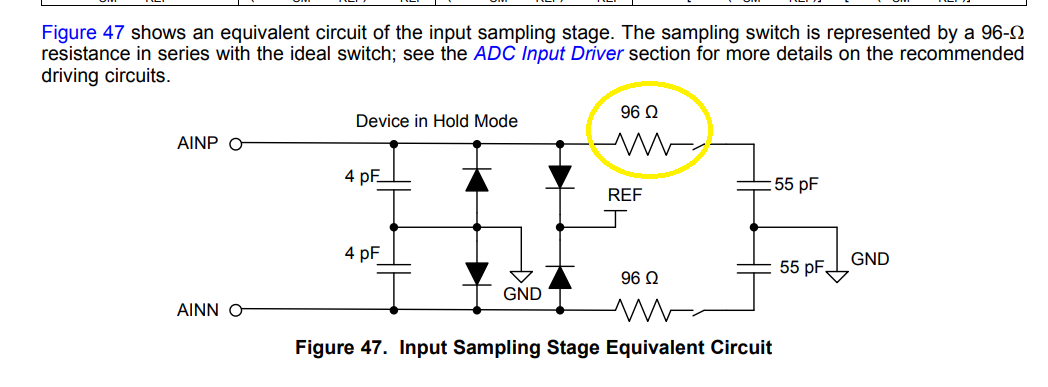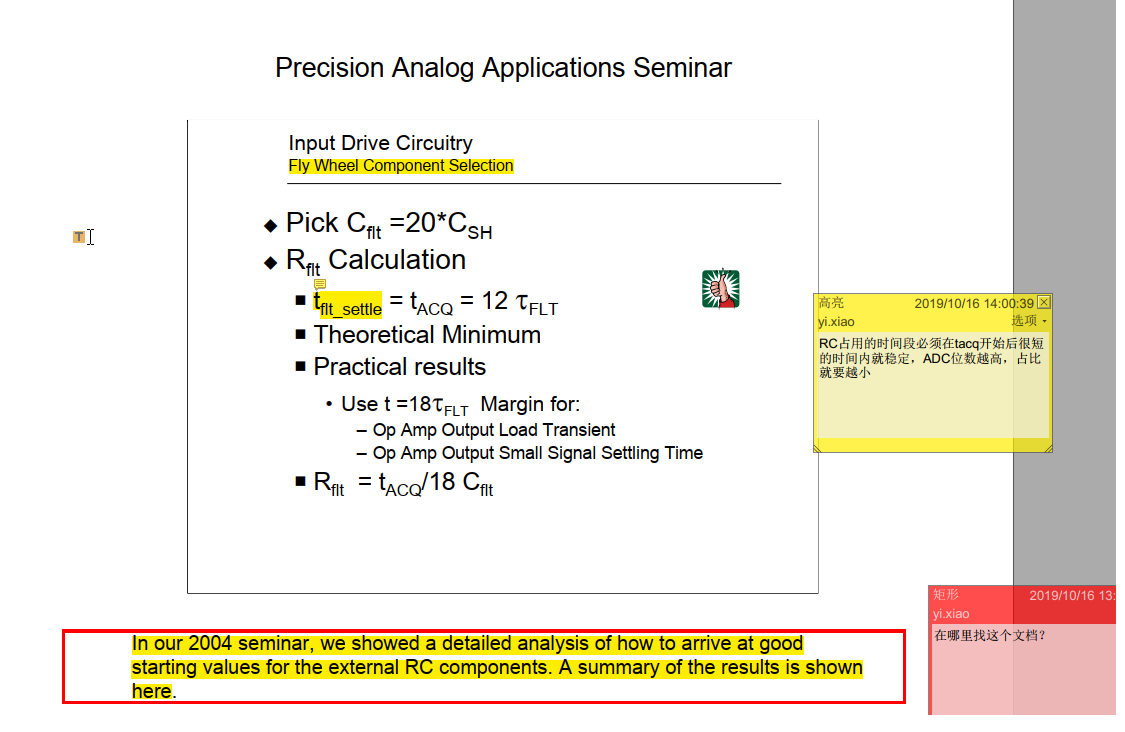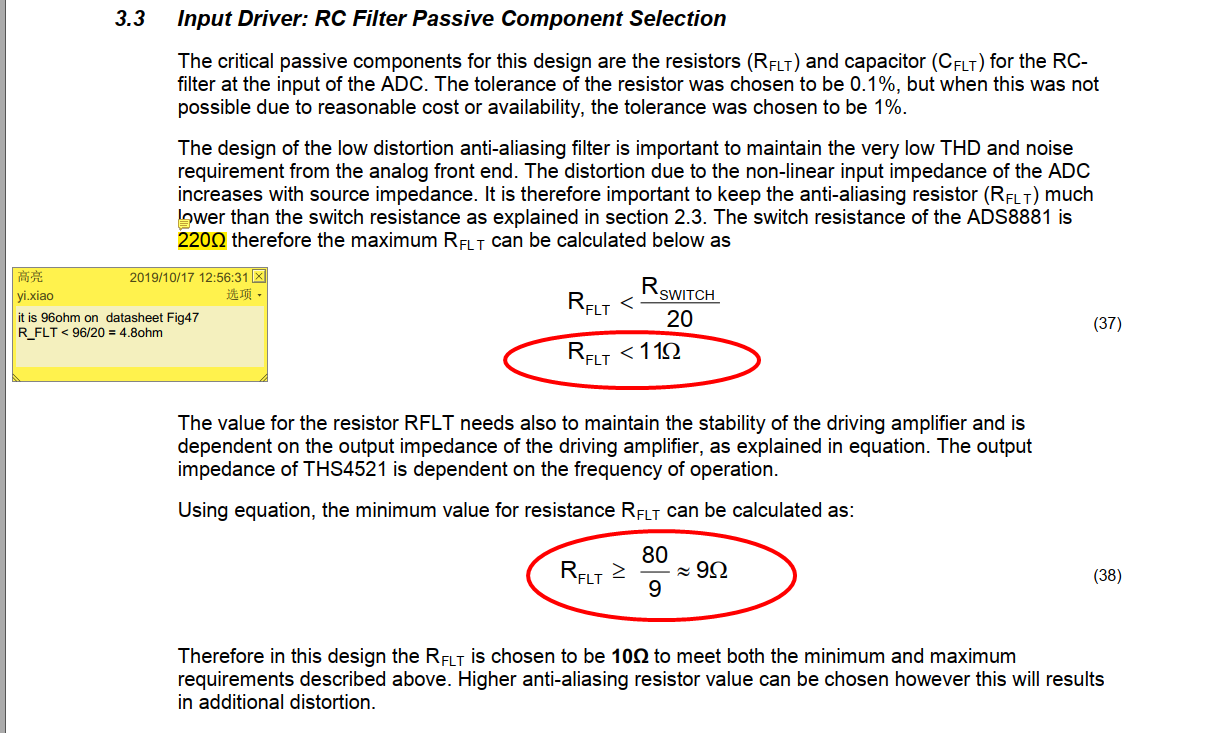This thread has been locked.

If you have a related question, please click the "Ask a related question" button in the top right corner. The newly created question will be automatically linked to this question.

# ADS8881: how to design RC filter for SAR ADC input.

Part Number: ADS8881
Other Parts Discussed in Thread: TIPD115, , THS4521

Hi there,

we are referring to TIPD115 and it design files (sch. pcb, etc) to design a quite  similar data acquisition system, we ues THS4521 to drive ADS8881 too .

When reading section about Anti-Alias-Filter , I have some questions. Hope some TI expert could help.

1. On page9 of  SLAU515A, it writes "output impedance of the driving amplifier is equal to Ro ". Does Ro refer to open-loop or close-loop output resistance of the amplifier? How do we get this value from amplifier's datasheet?

2. As in SCH, Ro seems to be  10ohm (R7 and R8) as below. Why equation (38) in SLAU515A take Ro=80ohm  ?3. Also in SLAU515A, equation (37), why R_switch of ADS8881 is 220ohm, I think it should be 96ohm as in ADS8881 datasheet.  Finally, I think  ti should be R_FLT < 96ohm/20 = 4.8ohm ,and R_FLT >10/9=1.1ohm.4. I noticed some other SAR ADC which does not provide R_switch value. what can we do with this situation ?

5. I also googled a very good tech note of TI on this SAR drive topic: "", it mentions on 2004 TI has senimar discussed this in details. Could you pls help where could I found this 2004 tech seminar PPT doc?Any other suggestion on this topic is appreciated.  Thanks very much!

• Hello Yi Xiao,

The 2004 seminar material has been updated and is now included in the TI Precision Labs - ADCs series.

https://training.ti.com/ti-precision-labs-adcs

You can watch videos, download presentations, and design files including TINA SPICE designs.

For specific information on how to select the RC values for the ADC input filter, please look at Section 5, SAR ADC Input Driver Design.

I recommend you download the Analog Engineer's Calculator, which has a section that calculates a range of RC values.

http://www.ti.com/tool/ANALOG-ENGINEER-CALC

Answers to your questions:

1. Ro refers to the open loop resistance of the amplifier. This can be found in Figure 43 of the THS4521 datasheet.
2. Ro refers to the internal open loop resistance of the amplifier, not external components. Per Figure 43, Ro varies with load capacitance. Since there is only parasitic board capacitance on the THS4521 outputs, 80ohm is an estimate assuming around 20pF of load capacitance.
3. ADS8881 R_switch resistance is 96ohm, not 220ohm. For calculation, please refer to the TI Precision Labs videos and the Analog Engineer's Calculator.
4. You can estimate the switch resistance. Please refer to Section 5.3 TI Precision Labs - ADCs: Building the SAR ADC Model
5. The older 2004 seminar material and the method used in the TI Precision Design has been updated and is now part of the TI Precision Labs - ADCs series.

Regards,

Keith Nicholas
Precision ADC Applications

• hi Keith, thanks for helping. I start to study the relevant part of those video , it will take some time. And for TI Analog Engineer's Calculator, I heard of this famous tool, and I often have a personal habbit to understand the math behind those tools before use them in practical design.

Here are some questions I hope to clear.

1. If "Ro refers to the internal open loop resistance of the amplifier", may I ask what's the usage of R7 and R8 (two 10ohm) serial resistor on the output of THS4521 in the SCH? As I understand, the amp need to have output impedance small enough to be able to drive a capacitive load.  And for AAF RC filter between amp and ADC, I thought it should be close-loop output resistance that actually matter, after all, we all use amp in close-loop form.

2.Since you confirmed ADS8881 has  96 ohm R_swtich, does it mean that the doc "SLAU515A" made some mistake? please see page 16 as sanpshot below, section 3.3, it is impossibe to find a R_FLT value which is both  >Ro/9 (9ohm) and < R_switch/20 (4.8ohm).

On the other hand, from the info I got in TI video “5.7 TI Precision Labs – ADCs: Math Behind the R-C Component Selection”， I think the key point is to keep RC time constant much smaller than t_acq of ADC. But the "R_FLT<R_switch/20" rules are not mentioned in videos, maybe this rule in doc SLAU515A is not a very reliable equation to determin max R_FLT value?Any insights from TI experts would be appreciated. Thanks very much.

• Hello Yi Xiao,

Answers to your questions.

1. You are correct. If the open loop output resistance of an amplifier is 0ohms, then it could easily drive a capacitive load. However, since every amplifier has a non-zero output resistance Ro, this resistance forms a pole in the frequency domain with the output load capacitance. It is the pole that causes amplifier instability. Also, the open loop Ro is not actually a resistance, but a complex impedance. Some amplifiers are very close to a pure resistance, but many are not. The THS4521 has an output impedance that is close to a resistor, but not exact.  Adding an additional 10ohms (R7 and R8) helps with the frequency compensation when dealing with an open loop, complex impedance. Take a look at the TI Precision Labs - Op Amps: Stability (Section 10) that discusses these concepts in much more detail. https://training.ti.com/ti-precision-labs-op-amps

1. The goal of the procedure in the TI Design (and the TI Precision Labs) is to set a range of values that should work. In both cases, you need to then build the circuit and measure proper operation in the lab. The TI Design was an older procedure that worked most of the time, but the new procedure in the TI Precision Labs has proven to be more accurate.   Please note that there are other RC combinations that can work for certain conditions. If you are sampling at a very low rate, for example, 10ksps, R_FLT could be much larger than the switch resistance.       Take a look at Figure 72 in the ADS8881 datasheet as an example.

Regards,
Keith

• Hi Keith, Thanks for helping. Here is another question on SPI design.

Now we use 3-wire CS mode without busy indicator. Single ADC connect to FPGA.

The conversion time ( t_conv ) is 500~710ns, determined by IC design, so we can not change it , and from chip to chip their t_conv time may distribute randomly among 500~710ns.

I think we need to keep CONVST signal HIGH time longer than 710ns  (like 750ns) to fully make ensure that conversion process is complete before we start to readback SDO data. As we need 1MSPS sample rate, there is only 250ns left for SPI readout 18bits, which means t_SLCK=250ns/18 = 13.89ns, so SCLK freq. = 72MHZ . That would exceed the spec 70MHZ.

Even if we change to use 3-wire CS mode with busy indicator, we can know the exact time when conversion complete, but the worst case still could be 710ns. so 290ns left, its on the margin of t_acq-min.

Do you have any suggestion? Thanks again.

• Hello Yi Xiao,

You are correct about observing the maximum conversion time of 710nS, but you do not need to wait more than 710nS.  Observing the setup time after CONVST goes low, and running the SCLK at 70MHz, you will have enough time to retrieve the data in the 290nS window, and still maintain the maximum throughput of 1Msps.  If you can sample at a slightly slower sample rate, this will increase the acquisition time, giving you more time to retrieve the data.

Regards,
Keith